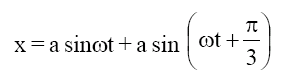Sample Paper Set II For 11th And 12th

15 Questions | Total Attempts: 253SettingsQuestion No: 1-5 (Physics) Question No: 6-10 (Chemistry) Question No: 11-15 (Mathematics)

• 1.
A charge q is placed at the centre of the line joining two equal charges Q. The system of the three charges will be in equilibrium if q is equal to :
• A.
• B.
• C.

• D.
• 2.
A hollow metal sphere is charged such that the potential at its centre is V. The potential on the surface of the sphere is :
• A.

Zero

• B.

V

• C.

More than V

• D.

Less than V

• 3.
An infinite number of charges, equal to q, are placed along the x-axis at x = 1, x = 2, x = 4, x = 8 .... and so on.The electric potential at the point x = 0 due to this set of charges is :
• A.
• B.
• C.
• D.
• 4.
The displacement of a particle executing simple harmonic motion varies with time t as The amplitude of oscillation is :
• A.

A

• B.
• C.
• D.

2 a

• 5.
Find the electric field due to three concentric thin long cylinders of linear charge densities –, +2 and just outside the outer cylinder.
• A.
• B.
• C.
• D.

None of these

• 6.
Reactivity of carbonyl compound towards NAR is-
• A.

III > I > II

• B.

III > II > I

• C.

I > II > III

• D.

I > III > II

• 7.
The ratio of total number of  bond to the total number of  bond in anhydride of
• A.

4

• B.

2

• C.

3

• D.

8

• 8.
Which of the following will have positively charged particles in dispersed phase?
• A.

sol

• B.

Milk

• C.

Blood

• D.

Gold sol

• 9.
Which of the following option is incorrect regarding ?
• A.
• B.

P–Cl bond length is less than P–F bond length.

• C.

Cl – – Cl bond angle is less than Cl – – F bond angle.

• D.

Its dipole moment is zero

• 10.
Aspartame, an artificial sweetener contains 9.52 wt. % nitrogen. There are two nitrogen atoms per molecule. What is the molecular weight of aspartame?
• A.

147

• B.

294

• C.

588

• D.

266

• 11.
There exists a natural number N which is 50 times its own logarithm to the base 10, then number of digits in N is
• A.

1

• B.

2

• C.

3

• D.

4

• 12.
• A.

1

• B.

–1

• C.
• D.
• 13.
The values of cos
• A.

0

• B.
• C.
• D.
• 14.
• A.

1

• B.

2

• C.

3

• D.

4

• 15.
Consider the function f(x) = Statement-1: f '() = 0. Statement-2: f(c) =
• A.

Statement-1 is true, statement-2 is true and statement-2 is correct explanation for statement- 1

• B.

Statement-1 is true, statement-2 is true and statement-2 is NOT the correct explanation for statement-1.

• C.

Statement-1 is true, statement-2 is false.

• D.

Statement-1 is false, statement-2 is true.

Related TopicsBack to top